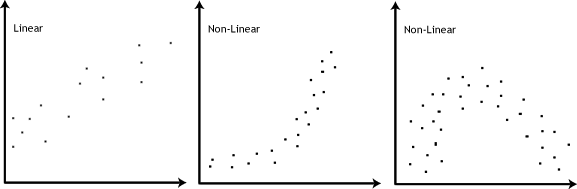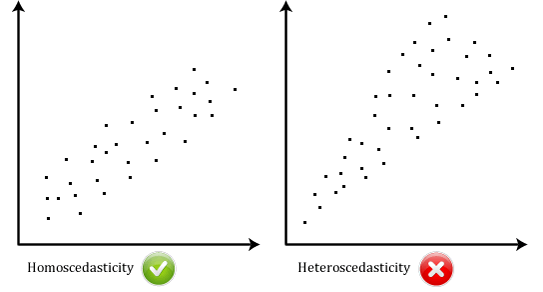# Linear RegressionThis article discusses the basics of linear regression and its implementation in Python programming language.

Linear regression is a statistical approach for modelling relationship between a dependent variable with a given set of independent variables.

Note: In this article, we refer dependent variables as response and independent variables as features for simplicity.

In order to provide a basic understanding of linear regression, we start with the most basic version of linear regression, i.e. Simple linear regression.

## Simple Linear Regression

Simple linear regression is an approach for predicting a response using a single feature.

It is assumed that the two variables are linearly related. Hence, we try to find a linear function that predicts the response value(y) as accurately as possible as a function of the feature or independent variable(x).

Let us consider a dataset where we have a value of response y for every feature x:For generality, we define:

x as feature vector, i.e$x = [x_1, x_2, ...., x_n]^T$,

y as response vector, i.e$y = [y_1, y_2, ...., y_n]^T$

for n observations (in above example, n=10).

A scatter plot of above dataset looks like:-Now, the task is to find a line which fits best in above scatter plot so that we can predict the response for any new feature values. (i.e a value of x not present in dataset)

This line is called regression line.

The equation of regression line is represented as:$y = [y_1, y_2, ...., y_n]^T$

Here,

•$h(x_i)$ represents the predicted response value for$i^{th}$ observation.
•$\beta_0$ and$\beta_1$ are regression coefficients and represent y-intercept and slope of regression line respectively.

To create our model, we must “learn” or estimate the values of regression coefficients$\beta_0$ and$\beta_1$. And once we’ve estimated these coefficients, we can use the model to predict responses!

In this article, we are going to use the Least Squares technique.

Now consider:$\beta_1$

Here,$\epsilon_i$ is residual error in$i^{th}$ observation.
So, our aim is to minimize$\sum_{i=1}^{n}\epsilon_i^2$.

We define the squared error or cost function, J as:$\sum_{i=1}^{n}\epsilon_i^2$

and our task is to find the value of$\beta_0$ and$\beta_1$ for which$J(\beta_0,\beta_1)$ is minimum!

Without going into the mathematical details, we present the result here:$J(\beta_0,\beta_1)$

where$SS_{xy}$ is the sum of cross-deviations of y and x:$SS_{xy}$

and$SS_{xx}$ is the sum of squared deviations of x:$SS_{xx}$

Note: The complete derivation for finding least squares estimates in simple linear regression can be found here.

Given below is the python implementation of above technique on our small dataset:

import numpy as np
import matplotlib.pyplot as plt

def estimate_coef(x, y):
# number of observations/points
n = np.size(x)

# mean of x and y vector
m_x, m_y = np.mean(x), np.mean(y)

# calculating cross-deviation and deviation about x
SS_xy = np.sum(y*x - n*m_y*m_x)
SS_xx = np.sum(x*x - n*m_x*m_x)

# calculating regression coefficients
b_1 = SS_xy / SS_xx
b_0 = m_y - b_1*m_x

return(b_0, b_1)

def plot_regression_line(x, y, b):
# plotting the actual points as scatter plot
plt.scatter(x, y, color = "m",
marker = "o", s = 30)

# predicted response vector
y_pred = b + b*x

# plotting the regression line
plt.plot(x, y_pred, color = "g")

# putting labels
plt.xlabel('x')
plt.ylabel('y')

# function to show plot
plt.show()

def main():
# observations
x = np.array([0, 1, 2, 3, 4, 5, 6, 7, 8, 9])
y = np.array([1, 3, 2, 5, 7, 8, 8, 9, 10, 12])

# estimating coefficients
b = estimate_coef(x, y)
print("Estimated coefficients:\nb_0 = {}  \
\nb_1 = {}".format(b, b))

# plotting regression line
plot_regression_line(x, y, b)

if __name__ == "__main__":
main()


Output of above piece of code is:

Estimated coefficients:
b_0 = -0.0586206896552
b_1 = 1.45747126437


And graph obtained looks like this:## Multiple linear regression

Multiple linear regression attempts to model the relationship between two or more features and a response by fitting a linear equation to observed data.

Clearly, it is nothing but an extension of Simple linear regression.

Consider a dataset with p features(or independent variables) and one response(or dependent variable).
Also, the dataset contains n rows/observations.

We define:

X (feature matrix) = a matrix of size n X p where$x_{ij}$ denotes the values of$j^{th}$ feature for$i^{th}$ observation.

So,$i^{th}$

and

y (response vector) = a vector of size n where$y_{i}$ denotes the value of response for$i^{th}$ observation.$i^{th}$

The regression line for p features is represented as:$i^{th}$

where$h(x_i)$ is predicted response value for$i^{th}$ observation and$\beta_0, \beta_1, ..., \beta_p$ are the regression coefficients.

Also, we can write:$\beta_0, \beta_1, ..., \beta_p$

where$\epsilon_i$ represents residual error in$i^{th}$ observation.

We can generalize our linear model a little bit more by representing feature matrix X as:$i^{th}$

So now, the linear model can be expressed in terms of matrices as:$i^{th}$

where,$i^{th}$

and$i^{th}$

Now, we determine estimate of$\beta$, i.e.$\hat{\beta}$ using Least Squares method.

As already explained, Least Squares method tends to determine$\hat{\beta}$ for which$\sum_{i=1}^{n}\epsilon_i^2$ is minimized.

We present the result directly here:$\sum_{i=1}^{n}\epsilon_i^2$

where$'$ represents the transpose of the matrix while$-1$ represents the matrix inverse.

Knowing the least square estimates,$\hat{\beta}$, the multiple linear regression model can now be estimated as:$\hat{\beta}$

where$\hat{y}$ is estimated response vector.

Note: The complete derivation for obtaining least square estimates in multiple linear regression can be found here.

Given below is the implementation of multiple linear regression technique on the Boston house pricing dataset dataset using Scikit-learn.

import matplotlib.pyplot as plt
import numpy as np
from sklearn import datasets, linear_model, metrics

# defining feature matrix(X) and response vector(y)
X = boston.data
y = boston.target

# splitting X and y into training and testing sets
from sklearn.model_selection import train_test_split
X_train, X_test, y_train, y_test = train_test_split(X, y, test_size=0.4,
random_state=1)

# create linear regression object
reg = linear_model.LinearRegression()

# train the model using the training sets
reg.fit(X_train, y_train)

# regression coefficients
print('Coefficients: \n', reg.coef_)

# variance score: 1 means perfect prediction
print('Variance score: {}'.format(reg.score(X_test, y_test)))

# plot for residual error

## setting plot style
plt.style.use('fivethirtyeight')

## plotting residual errors in training data
plt.scatter(reg.predict(X_train), reg.predict(X_train) - y_train,
color = "green", s = 10, label = 'Train data')

## plotting residual errors in test data
plt.scatter(reg.predict(X_test), reg.predict(X_test) - y_test,
color = "blue", s = 10, label = 'Test data')

## plotting line for zero residual error
plt.hlines(y = 0, xmin = 0, xmax = 50, linewidth = 2)

## plotting legend
plt.legend(loc = 'upper right')

## plot title
plt.title("Residual errors")

## function to show plot
plt.show()


Output of above program looks like this:

Coefficients:
[ -8.80740828e-02   6.72507352e-02   5.10280463e-02   2.18879172e+00
-1.72283734e+01   3.62985243e+00   2.13933641e-03  -1.36531300e+00
2.88788067e-01  -1.22618657e-02  -8.36014969e-01   9.53058061e-03
-5.05036163e-01]
Variance score: 0.720898784611


and Residual Error plot looks like this:In above example, we determine accuracy score using Explained Variance Score.
We define:$explained\_variance\_score = 1 - \frac{Var\{y - \hat{y}\}}{Var\{y\}}$
where$\hat{y}$ is the estimated target output,$y$ the corresponding (correct) target output, and$Var$ is Variance, the square of the standard deviation.
The best possible score is 1.0, lower values are worse.

## Assumptions

Given below are the basic assumptions that a linear regression model makes regarding a dataset on which it is applied:

• Linear relationship: Relationship between response and feature variables should be linear. The linearity assumption can be tested using scatter plots. As shown below, 1st figure represents linearly related variables where as variables in 2nd and 3rd figure are most likely non-linear. So, 1st figure will give better predictions using linear regression.• Little or no multi-collinearity: It is assumed that there is little or no multicollinearity in the data.  Multicollinearity occurs when the features (or independent variables) are not independent from each other.
• Little or no auto-correlation:  Another assumption is that there is little or no autocorrelation in the data.  Autocorrelation occurs when the residual errors are not independent from each other. You can refer here for more insight into this topic.
• Homoscedasticity: Homoscedasticity describes a situation in which the error term (that is, the “noise” or random disturbance in the relationship between the independent variables and the dependent variable) is the same across all values of the independent variables. As shown below, figure 1 has homoscedasticity while figure 2 has heteroscedasticity.As we reach to the end of this article, we discuss some applications of linear regression below.

## Applications:

1. Trend lines: A trend line represents the variation in some quantitative data with passage of time (like GDP, oil  prices, etc.). These trends usually follow a linear relationship. Hence, linear regression can be applied to predict future values. However, this method suffers from a lack of scientific validity in cases where other potential changes can affect the data.

2. Economics: Linear regression is the predominant empirical tool in economics. For example, it is used to predict consumption spending, fixed investment spending, inventory investment, purchases of a country’s exports, spending on imports, the demand to hold liquid assets, labor demand, and labor supply.

3. Finance: Capital price asset model uses linear regression to analyze and quantify the systematic risks of an investment.

4. Biology: Linear regression is used to model causal relationships between parameters in biological systems.